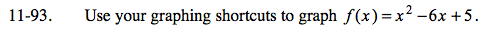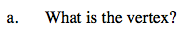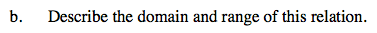### Home > CAAC > Chapter 11 > Lesson 11.2.2 > Problem11-93

11-93.Plot the x- and y-intercepts first. The y-intercept is given. To find the x-intercept,
substitute 0 for y and factor the quadratic. Plot the points you found.The vertex is exactly halfway between the two x-intercepts because a parabola's line of symmetry is on the vertex.

At the vertex, the x-coordinate is 3. Substitute 3 into the equation to solve for y and find the coordinates of the vertex.

Plot the point you found and sketch the parabola.What is the domain of this graph, that is, what are the possible x-values?

What is the range, or possible y-values, of this graph?

D: all real numbers R: y ≥ −4